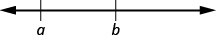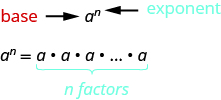## Key Concepts

Operation Notation Say: The result is…
Addition $a+b$ $a$ plus $b$ the sum of $a$ and $b$
Multiplication $a\cdot b,\left(a\right)\left(b\right),\left(a\right)b,a\left(b\right)$ $a$ times $b$ the product of $a$ and $b$
Subtraction $a-b$ $a$ minus $b$ the difference of $a$ and $b$
Division $a\div b,a/b,\frac{a}{b},b\overline{)a}$ $a$ divided by $b$ the quotient of $a$ and $b$
• Equality Symbol
• $a=b$ is read as $a$ is equal to $b$
• The symbol $=$ is called the equal sign.
• Inequality
• $a<b$ is read $a$ is less than $b$
• $a$ is to the left of $b$ on the number line• $a>b$ is read $a$ is greater than $b$
• $a$ is to the right of $b$ on the number lineAlgebraic Notation Say
$a=b$ $a$ is equal to $b$
$a\ne b$ $a$ is not equal to $b$
$a<b$ $a$ is less than $b$
$a>b$ $a$ is greater than $b$
$a\le b$ $a$ is less than or equal to $b$
$a\ge b$ $a$ is greater than or equal to $b$
• Exponential Notation
• For any expression ${a}^{n}$ is a factor multiplied by itself $n$ times, if $n$ is a positive integer.
• ${a}^{n}$ means multiply $n$ factors of $a$• The expression of ${a}^{n}$ is read $a$ to the $n$th power.

Order of Operations When simplifying mathematical expressions perform the operations in the following order:

1. Parentheses and other Grouping Symbols: Simplify all expressions inside the parentheses or other grouping symbols, working on the innermost parentheses first.
2. Exponents: Simplify all expressions with exponents.
3. Multiplication and Division: Perform all multiplication and division in order from left to right. These operations have equal priority.
4. Addition and Subtraction: Perform all addition and subtraction in order from left to right. These operations have equal priority.

## Glossary

expressions
An expression is a number, a variable, or a combination of numbers and variables and operation symbols.
equation
An equation is made up of two expressions connected by an equal sign.